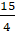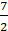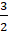Daily Practice Problems
Class 8 Maths
Linear Equations in One VariableQuestion 1:

Solve:– 7x = 9

Question 2:

The sum of a two-digit number and the number obtained by reversing its digits is 121. Find the number if its unit place digit is 5.

Question 3:

A steamer goes downstream from one point to another in 7 hours. It covers the same distance upstream in 8 hours. If the speed of stream be 2 km/h, find the speed of the steamer in still water and the distance between the ports.

Question 4:

Which of the following is not a linear equation in one variable?

A. 33z+5 = 0

B. 33(x + y) = 0

C. 33x+5 = 0

D. 33y+5 = 0

Question 5:

The age of the father is three times the age of the son. If the age of the son is 15 years old. Find the age of the father.

Question 6:

The difference between two whole numbers is 66. The ratio of the two numbers is 2: 5. Find both the numbers.

Question 7:

Three consecutive integers add up to 51. Find integers.

Question 8:

A fruit seller buys some oranges at the rate of ₹ 5 per orange. He also buys an equal number of bananas at the rate of ₹ 2 per banana. He makes a profit of 20% on oranges and a profit of 15% on bananas. In the end, he sold all the fruits. If he earned a profit of ₹ 390, find the number of oranges.

Question 9:

The sum of two numbers is 2490. If 6.5 % of one number is equal to 8.5 % of the other. Find the numbers.

Question 10:

Solve for x:

(a) (3x-5) = (x+ 5)

(b) (9x – 3) = (7x + 3)

Question 11:

The perimeter of a rectangle is 40 cm. If its width is 10 cm, then find the length.

Question 12:

The difference between two positive numbers is 40 and the ratio of these integers is 1: 3. Find the integers.

Question 13:

The angles of a triangle are in the ratio 2: 3: 4. Find the angles of the triangle.

Question 14:

Deveshi has a total of Rs 590 as currency notes in the denominations of Rs 50, Rs 20 and Rs10. The ratio of the number of Rs 50 notes and Rs 20 notes is 3:5. If she has a total of 25 notes, how many notes of each denomination she has?

Question 15:

The largest number of the three consecutive numbers is x + 1. Then, find the smallest number.

Question 16:

Arjun is twice as old as Shriya. Five years ago, his age was three times Shriya’s age. Find their present ages.

Question 17:

5x +=x -14

Question 18:

The digits of a two-digit number differ by 3. If the digits are interchanged, and the resulting number is added to the original number, we get 143. What can be the original number?

Question 19:

Present ages of Anu and Raj are in the ratio 4:5. Eight years from now the ratio of their ages will be 5:6. Find their present ages.

Question 20:

The difference between two whole numbers is 66. The ratio of the two numbers is 2: 5. What are the two numbers?

***********